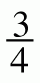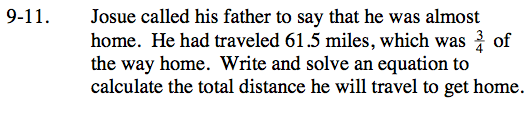### Home > CC3 > Chapter Ch9 > Lesson 9.1.1 > Problem9-11

9-11.
1. Josue called his father to say that he was almost home. He had traveled 61.5 miles, which wasof the way home. Write and solve an equation to calculate the total distance he will travel to get home. Homework Help ✎Let x represent the total distance to home.

$\frac{3}{4}\; \text{of the total distance is 61.5 miles.}$

How can you represent this as an equation?

$\frac{3}{4}{\it x} = 61.5$

What does x equal?